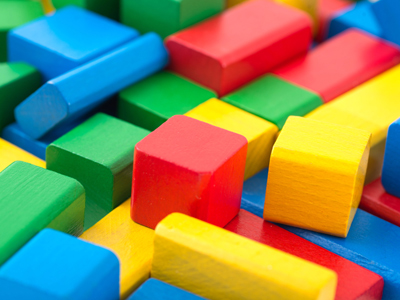What do you know about volume? Find out in this enjoyable quiz.

# Volume

This Math quiz is called 'Volume' and it has been written by teachers to help you if you are studying the subject at high school. Playing educational quizzes is a user-friendly way to learn if you are in the 9th or 10th grade - aged 14 to 16.

It costs only \$12.50 per month to play this quiz and over 3,500 others that help you with your school work. You can subscribe on the page at Join Us

In the same way that AREA tells us how much space a two-dimensional shape takes up, VOLUME is all about how much space a three-dimensional space needs. 2-D shapes can be drawn on a flat sheet of paper, whilst 3-D shapes have an extra dimension. Take this high school Math quiz and see if you have the extra dimension!

Volume is closely linked with CAPACITY, which is how much fluid a container can hold. Whilst volume is measured in units like m3 and cm3, capacity is measured in liters, ml and so on. You should be able to work between the two systems, the key is 1m3 holds 1,000 liters. Did you know, 1m3 of water weighs a ton? That’s heavy!

The volume of cubes and cuboids is straightforward to calculate, it’s just length x base x height.

But most shapes you will come across aren’t so easy. Many of them will be prisms – 3-D objects that have 2 opposite faces of the same size and shape, joined by a series of rectangles. A cylinder is an example of a prism. They are usually named by the shape of the face, so a cylinder could also be called a circular-based prism.

The volume of a prism is the area of the face multiplied by its length. You are normally given this formula in the high school exam paper, but make sure you know how to use it!

Question 1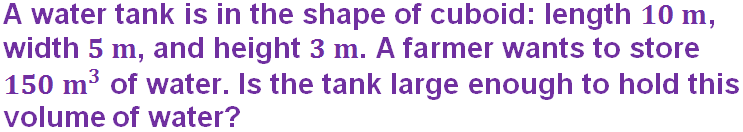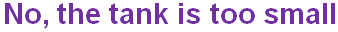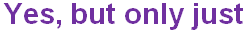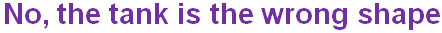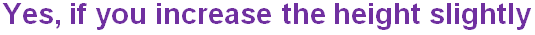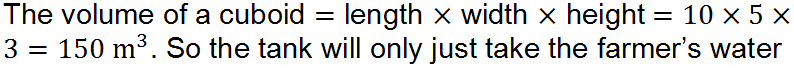Question 2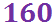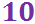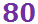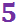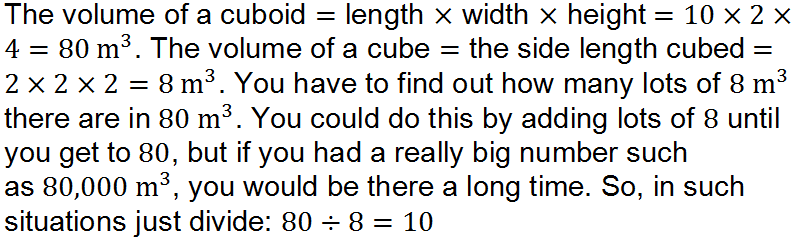Question 3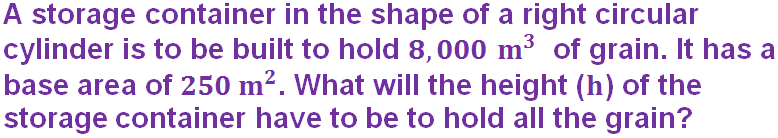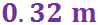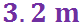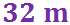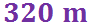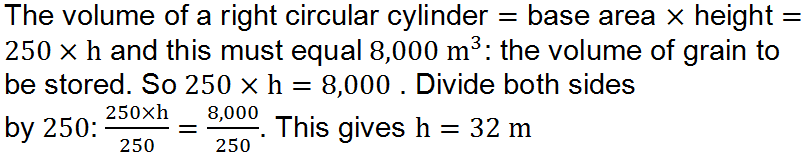Question 4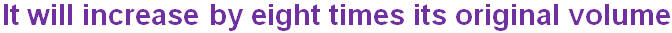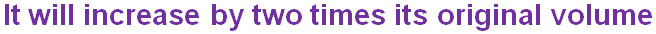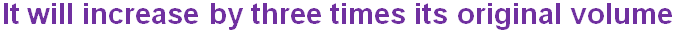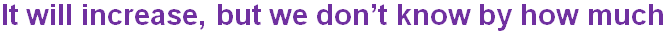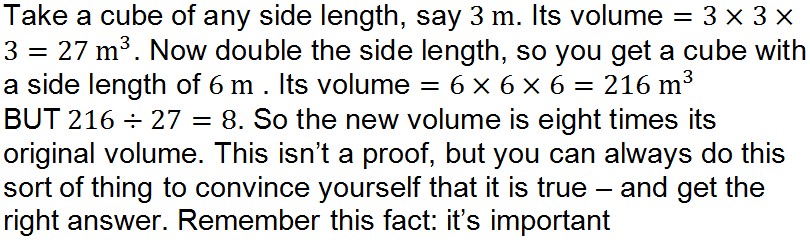Question 5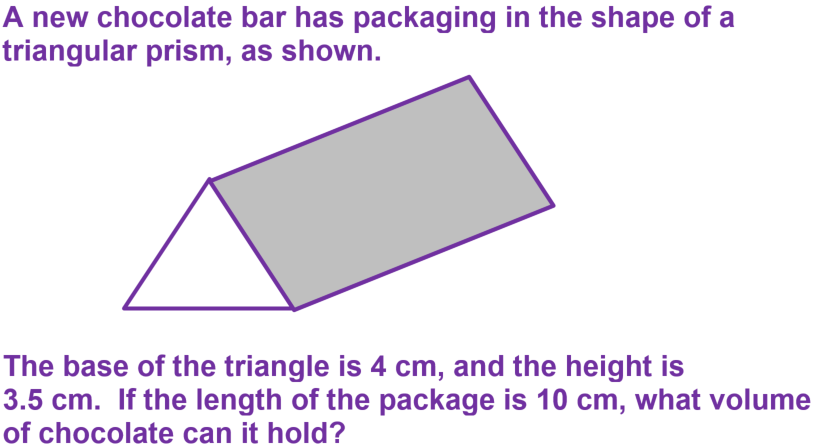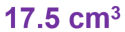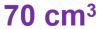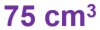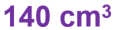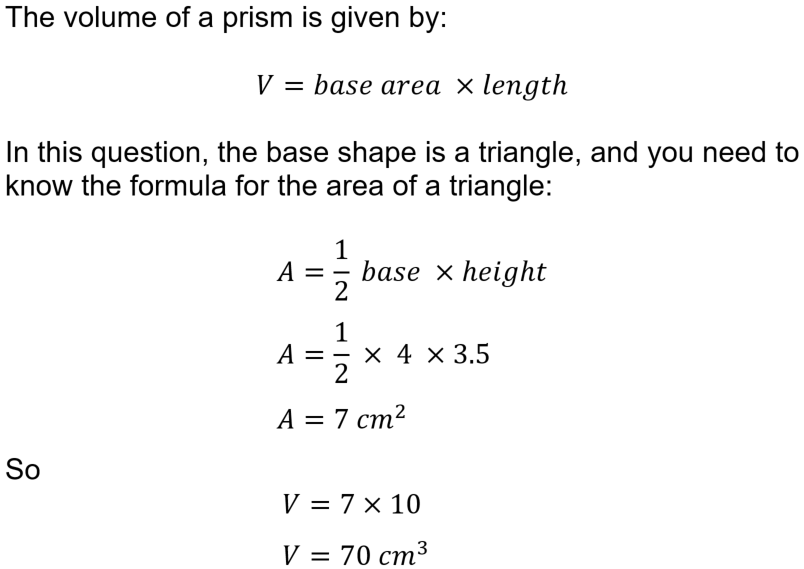Question 6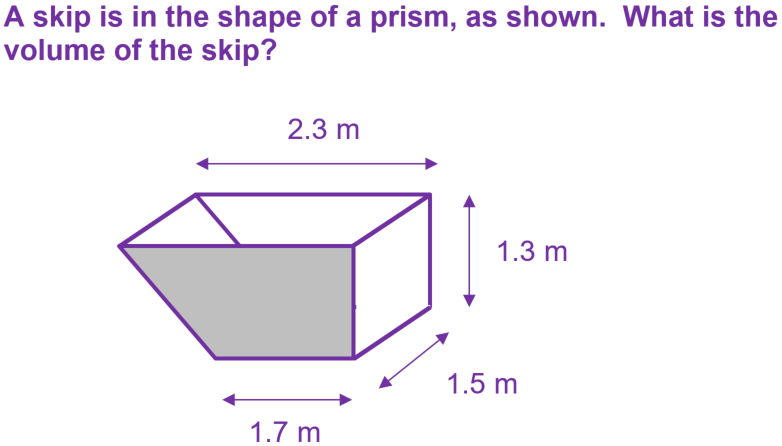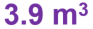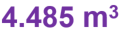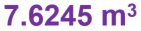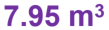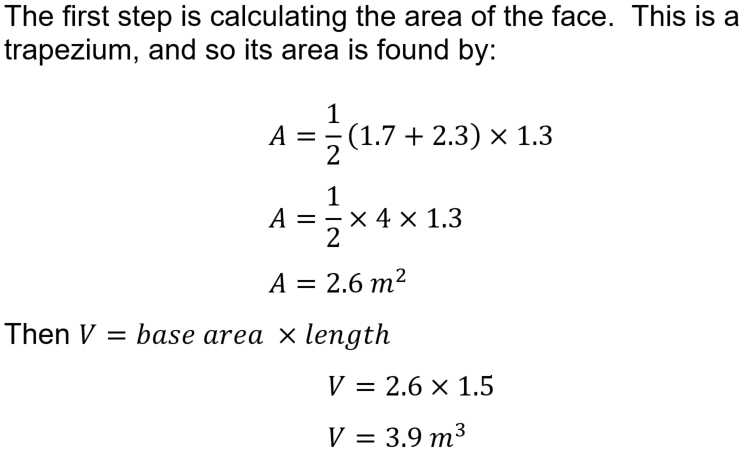Question 7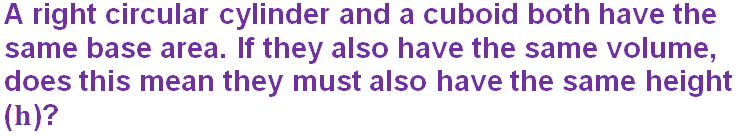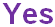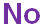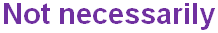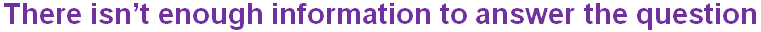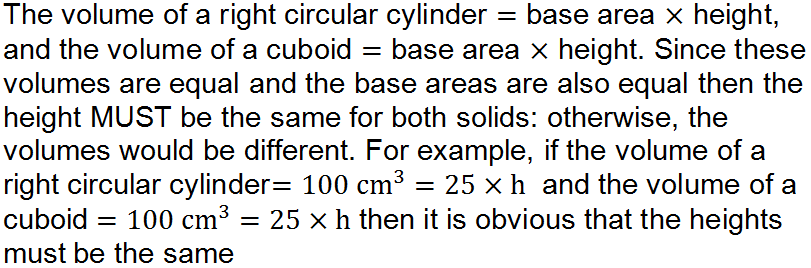Question 8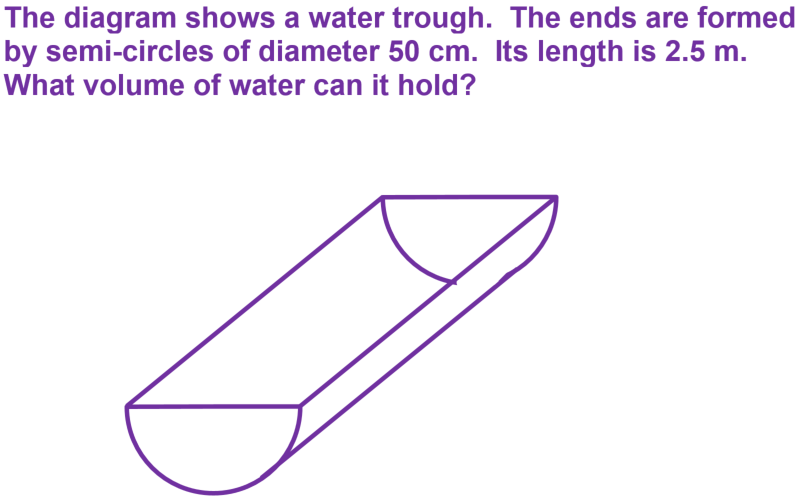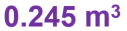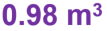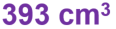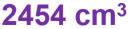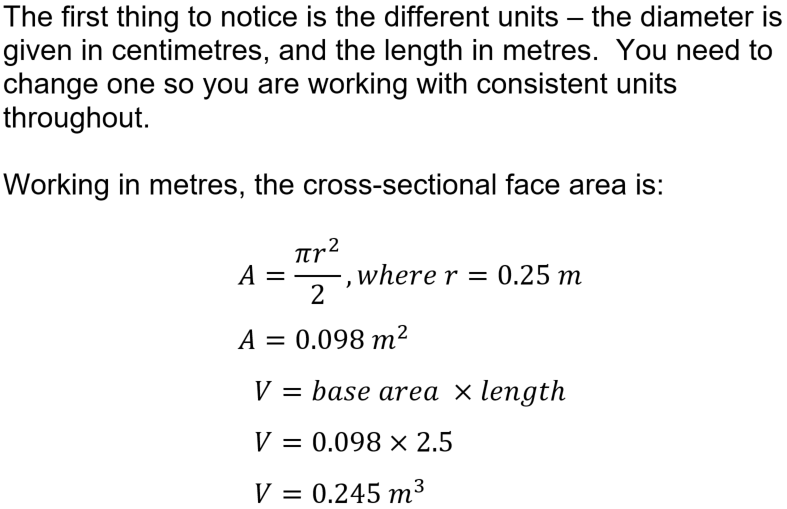Question 9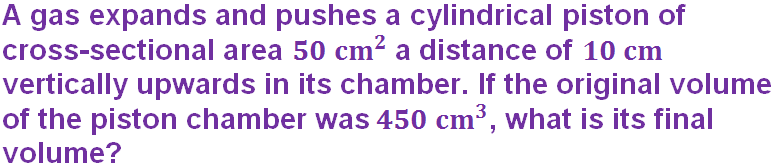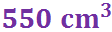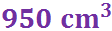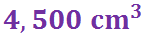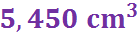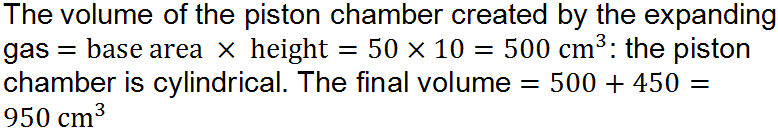Question 10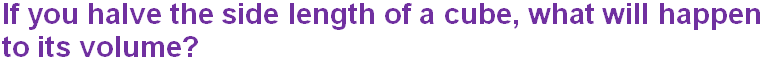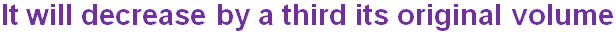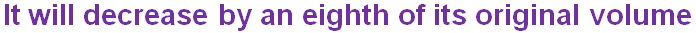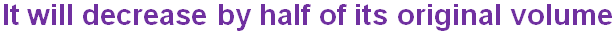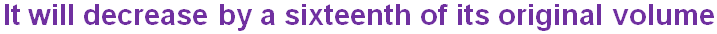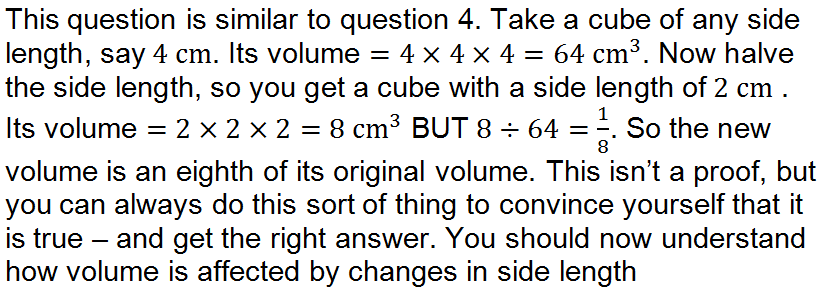Author:  Frank Evans